# How to Create a Pivot table with multiple indexes from an excel sheet using Pandas in Python?

The term Pivot Table can be defined as the Pandas function used to create a spreadsheet-style pivot table as a DataFrame. It can be created using the pivot_table() method.

Syntax: pandas.pivot_table(data, index=None)

Parameters:

data : DataFrame
index: column, Grouper, array, or list of the previous

index: It is the feature that allows you to group your data.

Returns: DataFrame

Note: We can filter the table further by adding the optional parameters.

Example 1: Link to the CSV File: CSV FILE
We can have a look at the data by running the following program:

## Python3

 `# importing pandas as pd  ` `import` `pandas as pd ` ` `  `# Create the dataframe  ` `df``=``pd.read_csv(``'GeeksForGeeks.csv'``) ` ` `  `# Print the dataframe  ` `df `

Output: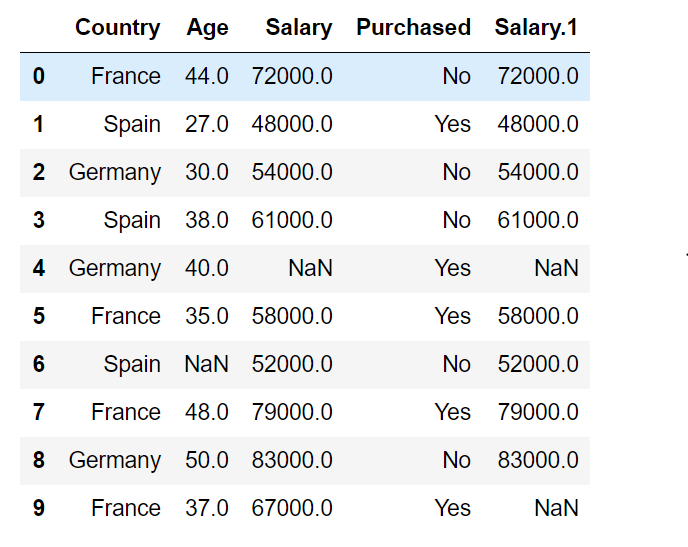We know that the index is the feature that allows us to group our data and specifying multiple columns as the indices in pivot function increases the level of details and grouping the data.
Keeping a single index in the table:

## Python3

 `# importing pandas as pd  ` `import` `pandas as pd ` ` `  `# Create the dataframe  ` `df``=``pd.read_csv(``'GeeksForGeeks.csv'``) ` ` `  `# Print the resultant table ` `print``(pd.pivot_table(df,index``=``[``"Country"``])) `

Output: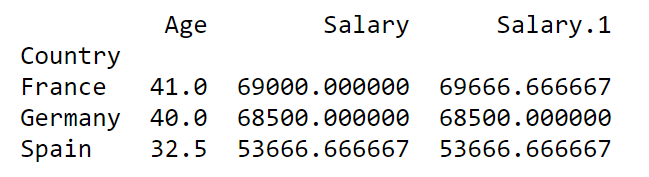As we can see that the grouping is done country wise and the numerical data is printed as the average of all the values with regard to the specified index.
Now, Keeping multiple indices in the table:

## Python3

 `# importing pandas as pd  ` `import` `pandas as pd ` ` `  `# Create the dataframe  ` `df``=``pd.read_csv(``'GeeksForGeeks.csv'``) ` ` `  `# Print the resultant table ` `print``(pd.pivot_table(df,index``=``[``"Country"``,``"Salary"``])) `

Output: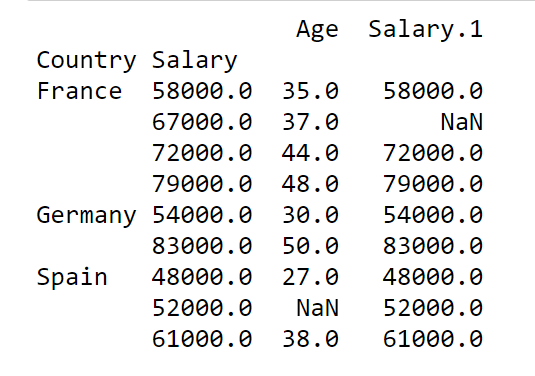Example 2: Link to the CSV File: CSV FILE

## Python3

 `# importing pandas as pd  ` `import` `pandas as pd ` ` `  `# Create the dataframe  ` `df``=``pd.read_csv(``'GeeksForGeeks_1.csv'``) ` ` `  `# Print the dataframe  ` `df `

Output: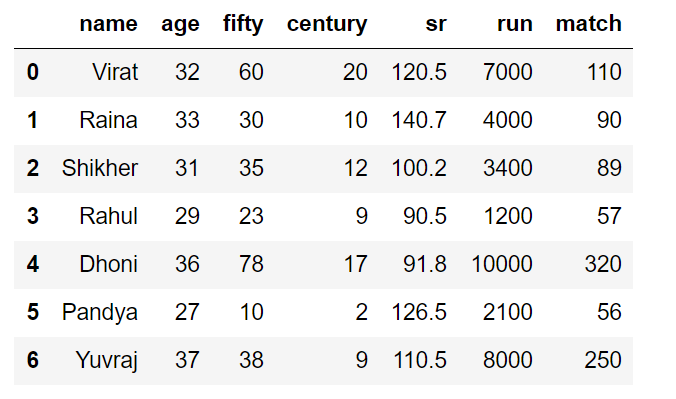Keeping the number of centuries scored by players and their names as indices, we get:

## Python3

 `# importing pandas as pd  ` `import` `pandas as pd ` ` `  `# Create the dataframe  ` `df``=``pd.read_csv(``'dataset/new_players.csv'``) ` ` `  `# Print the resultant table ` `print``(pd.pivot_table(df,index``=``[``"century"``,``"name"``])) `

Output: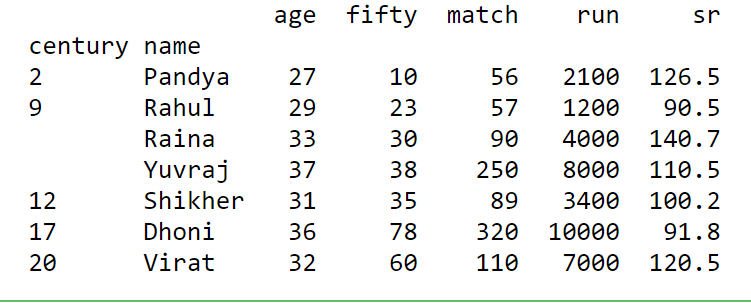My Personal Notes arrow_drop_upIf you like GeeksforGeeks and would like to contribute, you can also write an article using contribute.geeksforgeeks.org or mail your article to contribute@geeksforgeeks.org. See your article appearing on the GeeksforGeeks main page and help other Geeks.

Please Improve this article if you find anything incorrect by clicking on the "Improve Article" button below.

Article Tags :

1

Please write to us at contribute@geeksforgeeks.org to report any issue with the above content.